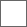### Flash动画笔记

1.文字特效：（功能菜单显示的前提是两个关键帧）
A 翻转文字：1.留底版，打散 开（ctrl+b），转原件，分散层.
B分裂文字：滤镜模糊和透明度
2金字塔变色：A调渐变（渐变类型）B第二祯-修改-变形-翻转。C修改ˉ形状ˉ添加形状提示。（按ctrl）
3曲线运动：A被引导层必须是原件，引导层必须是唯一的两个端点。B球进圈

1影片剪辑的二次转元：同一原件，整体调用。2遮罩动画：a属性必须是填充b窟窿外由背景控制c一对多（放大镜白底黑字两层）

1.关键帧
2.按钮
3.影片剪辑

1.添加在关键帧上的脚本（控制动画流程即控制时间轴）

2.添加在按钮和影片剪辑上（控制按钮和影片剪辑）

play() 播放
stop() 停止
gotoAndPlay() 跳转并播放
gotoAndStop() 跳转并停止
nextFrame() 下一帧
preveFrame() 上一帧

on(触发条件){执行语句}

release 松开
rollover 滑过
rollout 滑离
dragover 拖动滑过
dragout 拖动滑离

onClipEvent(触发条件){执行语句}

mousedown 鼠标按下
mousemove 鼠标移动
enterframe控制当前影片剪辑所在的每一帧中的当前影片剪辑
mouseup 鼠标松开

=变量赋值 i=1
==数学意义上的等号 1+1==2
===恒等于

()作用：1.系统自带的无任何意义play()
2.设置条件或属性或值
{}作用：设置具体执行语句

i = random(100); 随机函数
duplicateMovieClip("a", "a" + i, i);母体 字体 深度
setProperty("a" + i, _x, random(500));字体 属性 值
setProperty("a" + i, _y, random(300) + 100);
setProperty("a" + i, _xscale, i);
setProperty("a" + i, _yscale, i);

if (i < 25)
{
duplicateMovieClip("aa", "aa" + i, i);
setProperty("aa" + i, _rotation, i * 5);
setProperty("aa" + i, _x, _root._xmouse);
setProperty("aa" + i, _y, _root._ymouse);
++i;
}
else
{
i = 1;
} // end else if

for (i = 1; i < 10; i++)
{
setProperty("aa" + i, _rotation, random(30) - 10);
setProperty("aa" + i, _xscale, random(50) - 10);
setProperty("aa" + i, _yscale, random(50) - 10);
} // end of for

i = random(100);
duplicateMovieClip("a", "a" + i, i);
setProperty("a" + i, _x, random(550));
setProperty("a" + i, _y, random(400))

new Color(a).setRGB(random(16777200));
if (i < 30)
{duplicateMovieClip("a", "a" + i, i);
setProperty("a" + i, _alpha, 100 - i * 5);
}
else
{
stop ();
}
++i;

new Color(this).setRGB(random(16777215));_root.aa._visible = 0;隐藏母体
for (i = 1; i < 50; i++)
{
duplicateMovieClip("aa", "aa" + i, i);
setProperty("aa" + i, _x, "aa");
setProperty("aa" + i, _y, "aa");
setProperty("aa" + i, _rotation, i * 1);
} // end of for

on (press) {
i++;
duplicateMovieClip("aa", "aa" + i, i);
setProperty("aa" + i, _x, random(300));
new Color("aa" + i).setRGB(random(0xffffff));
}attachMovie正确代码（一帧调用完成）
i=-1
n=10
onEnterFrame=function()
{i++;n++
if(i<10)
{attachMovie("aa","aa"+i,i,{_x=200,_y=200,_rotation:10*i})
attachMovie("nn","nn"+i,i,{_x=200,_y=200,_rotation:-10*i}
}
else
{stop()}
}
attachMovie错误代码
i=-1
n=10
onEnterFrame=function()
{i++;n++
if(i<10)
{attachMovie("aa","aa"+i,i,{_x:200,_y:200,_rotation:10*i});
attachMovie("aa","aa"+n,n,{_x:200,_y:200,_rotation:-10*i});
}
else
{stop()}
}
duplicateMovieClip正确代码（两个图层三针完成）
if (i < 25)
{
duplicateMovieClip("aa", "aa" + i, i);
setProperty("aa" + i, _rotation, i * 5);

i++;
}
else
{
i = 1;
}
if (i < 25)
{
duplicateMovieClip("aa", "aa" + i, i);
setProperty("aa" + i, _rotation, i *- 5);

i++;
}
else
{
i = 1;
}onEnterFrame = function ()
{
i = random(100);
duplicateMovieClip("aa", "aa" + i, i);
setProperty("aa" + i, _x, random(550));
setProperty("aa" + i, _y, random(400));
new Color("aa"+i).setRGB(random(0xffffff))
};

i = 1;
onEnterFrame = function ()
{
if (i <25)
{ i++
duplicateMovieClip("aa", "aa" + i, i);
setProperty("aa" + i, _x, _xmouse);
setProperty("aa" + i, _y, _ymouse);
}
else
{
i=1
}
};

i = 1;
bb.onPress = function()
{
i++;
duplicateMovieClip("aa", "aa" + i, i);
setProperty("aa" + i, _y, random(700));
new Color("aa"+i).setRGB(random(0xffffff))
};setProperty("aa" + i, _y, random(400))

var mysound:Sound=new Sound() // 新建声音容器才 var声明变量 空格 mysound变量名称 冒号 Sound变量类型 等号
mysound.attachSound("aa")//容器中绑定声音的链接名
mysound.start(50,1)//设置声音播放开始的帧数和播放次数

var mysound:Sound=new Sound() // 新建声音容器才 var声明变量 空格 mysound变量名称 冒号 Sound变量类型 等号
mysound.start(100,1)//设置声音播放开始的帧数和播放次数

var music:Sound = new Sound();

music.onSoundComplete = function()
{
music.start();
};

for (i = 0; i < 4; i++)
{
attachMovie("aa","aa" + i,i,{_x:i * 100, _y:200});
var kk:Array = new Array("首页", "产品", "售后", "服务");
_root["aa" + i].bb.text = kk[i];
}

i = 0x000000;
n = 2;
onEnterFrame = function ()
{
lineStyle(n,i,100);
};
onMouseDown = function ()
{
moveTo(_xmouse,_ymouse);
onMouseMove = function ()
{
lineTo(_xmouse,_ymouse);
};
onMouseUp = function ()
{
delete onMouseMove;
};
};
aa.onPress = function()
{
i = 0xff0000;
};
bb.onPress = function()
{
n = 10;
};
cc.onPress = function()
{
i = 0xffffff;
n = 50;
};

#### 扫二维码下载贴吧客户端